Keroscene / 453 Project
Keroscene
Keroscene was the raytracer I wrote for CPSC 453 (Graphics I). The program included several types of primitives, including spheres, cylinders, cones, and triangles. Scaling spheres allowed for the creation of ellipsoids. Reflection, Refraction, and Shadows were implemented. Distributed ray tracing was applied to circular light sources, allowing for soft shadows (umbra/penumbra). Jittered anti-aliasing, solid texturing and CSG subtraction were also implemented. Several types of light sources were added, including infinite lights and spotlights with cone controls.
Keroscene Images

The left image below is my favorite (it also won the class contest for best image). I used just about every feature of my raytracer for that one - there is CSG subtraction, spotlights with cone control, reflection, refraction, solid textures, and it's well anti-aliased (though jpeg compression adds some artifacts). The only thing missing was soft shadows. You might notice that the spheres are a little distorted, especially in the reflections near the bottom of the image. I had the field of view set wrong. Click for a larger view. The simple right image was an in-progress shot.

The three images below show (left to right) a solid textured sphere, the same sphere (differnt color) with some CSG subtraction, and a scene with lots of csg and soft shadows.

Finally, I played with the index of refraction for a transparent sphere.

Generalised Cylinders

Generalised Cylinders are amazing. Take a Bezier or B-Spline curve. Now generate cross-sections perpendicular to the curve at constant intervals. Connect these sections together. Voila, a cylinder that follows the curvature of your parametric curve. Now, instead of using a constant radius for the sections, define another curve and use it to vary the radius of the sections. Like magic. I wrote a basic curve editor that generated the images below. Click on them for larger versions.

Directed Acylicic Graphs (DAGs)

Directed Acylic Graphs are used to describe heirarchial models of objects. The name is sort of misleading, because the graphs I used to generate the pictures below are actually cyclic...

Here's a tree DAG at recursion limits 2, 7, and 10.

I call the one on the left Hippopotamonkey. The alpha blending in the left image doesn't look so good (thanks, SGI). The one on the right is Hardihosh.

L-Systems and Space-Filling Curves

L-Systems (Lindenmeyer Systems) are nifty. They can be described by DAGs, but these simple L-Systems shown below are just described by strings of instructions (turn left, turn right, move forward). These instructions can be used to describe Space-Filling Curves (SFC's). Space-Filling curves will touch every point in a grid of whatever shape they fill. They also look neat.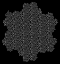Peano-Gosper curve: Axiom: XF Angle: 60 degrees X: X+YF++YF-FX--FXFX-YF+ Y: -FX+YFYF++YF+FX--FX-Y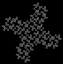32 Segment curve: Axiom: F+F+F+F Angle: 90 degrees F: -F+F-F-F+F+FF-F+F+FF+F-F-FF+ FF-FF+F+F-FF-F-F+FF-F-F+F+F-F+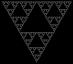Arrowhead curve: Axiom: YF Angle: 60 degrees X: YF+XF+Y Y: XF-YF-X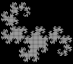Dragon curve: Axiom: FX Angle: 90 degrees X: X+YF+ Y: -FX-Y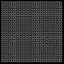Hilbert curve: Axiom: L Angle: 90 degrees L: +RF-LFL-FR+ R: -LF+RFR+FL-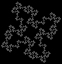Koch Island: Axiom: F+F+F+F Angle: 90 degrees F: F-F+F+FFF-F-F+F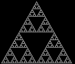Sierpinksi Triangle: Axiom: FXF--FF--FF Angle: 60 degrees F: FF X: --FXF++FXF++FXF--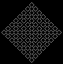Square curve: Axiom: F+XF+F+XF Angle: 90 degrees X: XF-F+F-XF+F+XF-F+F-X

 Go fish? mail rms@unknownroad.com.